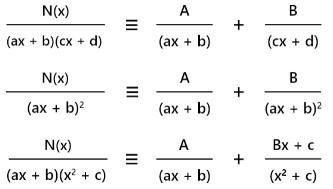# Partial Fraction Calculator

To find rational fraction, enter function and click calculate button using partial fraction calculator

This will be calculated:

Give Us Feedback

## Partial Fraction Calculator

Partial fraction calculator is used to expand the polynomial rational functions with steps. This partial fraction decomposition calculator takes the numerator and denominator of a function to decompose that function.

## What is the partial fraction?

In mathematics, a partial fraction is a method to write a rational function (quotient of two polynomials) as the sum of simpler rational expressions. It is also written as the sum of the fractions whose denominators are the linear binomials.

### Formulas of partial fraction## How to calculate the partial fraction?

Here is a solved example of the partial fraction.

Example

Find the partial fraction of (2x2 + 1)/(x+1)(x+5)

Solution

Step 1: Write the formula of the partial fraction.

F(x)/(ax+b)(cx+d) = A/(ax+b) + B/(cx+d)

Step 2: Substitute the given rational function.

(2x2 + 1)/(x+1)(x+5) = A/(x+1) + B/(x+5) … equation (1)

Step 3: Multiply by (x+1)(x+5) on both sides of the above expression.

(x+1)(x+5) * (2x2 + 1)/(x+1)(x+5) = (x+1)(x+5) * A/(x+1) + (x+1)(x+5) * B/(x+5)

(2x2 + 1) = A(x+5) + B(x+1)

Step 4: Put x = -1 in the above expression.

(2(-1)2 + 1) = A(-1+5) + B(-1+1)

(2(1) + 1) = A(4) + B(0)

(2 + 1) = 4A + 0

3 = 4A

A = 3/4

Step 5: Now Put x = -5.

(2(-5)2 + 1) = A(-5+5) + B(-5+1)

(2(25) + 1) = A(0) + B(-4)

(50 + 1) = 0 - 4B

51 = - 4B

B = -51/4

Step 6: Put the values of A & B in equation (1)

(2x2 + 1)/(x+1)(x+5) = 3(x+1)/4 - 51(x+5)/4

### Math Tools